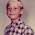### Pet peeves with Common Core Standards, part 2

QUOTE from Common Core standards for 7th grade:

The Number System 7.NS
c. Show that the distance between two rational numbers on the number line is the absolute value of their difference, and apply this principle in real-world contexts.

Okay, I am not with this totally.

For example, let's say you are supposed to find the distance between −4/7 and −5/9. Two negative fractions. They want the student to construct the difference −4/7 − (−5/9) and then find its absolute value. Remember we are in 7th grade.

I find a more natural approach (and isn't it easier to SHOW this to students?) is to find the distance between 4/7 and 5/9, which is of course equal to the distance between −4/7 and −5/9.

I feel students might have difficulties if they are supposed to take the difference of two negative numbers, and find the absolute value of that. They might make mistakes even in writing out the expression −4/7 − (−5/9) correctly.

ALSO, the standard actually words it as "SHOW that...". In other words, IF I understand this correctly, students aren't only supposed to know how to find the distance, but also to be able to PROVE that "the distance between two rational numbers on the number line is the absolute value of their difference."

In mathematical symbols, do they really want the STUDENTS to show/prove that

The distance of p and q = | p − q| , for all rational numbers p and q.

When it gets to dealing with negative fractions & decimals, I feel it would be enough for 7th graders to show INFORMALLY, using a number line, HOW to find the distance, without having to write it with symbols (where you need to use the absolute value OF the difference).

I mean exercises such as these:
• Explain how to find the distance between -0.8 and 4.9.
• How far apart are −1/2 and −1 1/4?
• Explain how to find the distance between −200 and −1,600.

Any thoughts?Angie said…
Oh boy, you lost me there. Could we get a video presentation on this or something ?Stephen said…
My 6th and 8th graders get these sorts of questions. I expect that some of it has to do with what the students are being taught. Geometry (9th grade for me) had proofs all the time. They weren't that hard. We were taught how to do them. It's quite clear to me that my 6th and 8th grade students aren't being taught this. It's not in their books. So what is this about? Is it a question that the teacher feels they can use to give some kids an A, and others a B?

When my 6th grader was in 4th grade, they had "problem of the week". These weren't graded, but were, roughly speaking, impossible. Add the numbers from 1to 100. Simple. It's 5,050. Just bang it out on a calculator. Opps, pressed a wrong button, so start over. Opps, ran out of attention span. Or, just go to a computer and type gcc < "main() { int i, s; s=0; for (i = 1; i <= 100; i++) s+=i; printf("%d\n", s);}"
Then "./a.out". (Sorry for any typos.)

Or, use the closed form x*(x+1)/2, for x=100. These approaches are beyond most 4th graders.

Gauss was said to have solved this in first grade, in a minute or so. I doubt it. He's said to have said that 1 + 100 is 101. 2 + 99 is 101. The numbers meet at 50 + 51, which is 50 numbers. So 50 * 101 = 5050. But i learned multiplication in 4th grade. I think it's taught a bit earlier now, but not in 1st grade.Most of these standards are very difficult to interpret without examples. Now I am a science teacher, but we do lots of math too so here is my thought. Like most things I think the focus should be on the "real world" part of the standard. A good example being your in a basement 3m underground and you mom is on the second floor 7.7m above the ground how far apart are you and your mom (draw a number line representing you and your mother). I think it is important to use situations that kids might be familiar with so they get the concept without getting hung up on a scenario they dont understand. And I dont think "show" means "prove" as you described i think it means draw a number line showing informally as you stated the distance between two points similiar to my example. The key perhaps is the absense of the word "any" as in "show the distance between ANY two rational numbers" which would imply a formal proof. Hope I made sense.